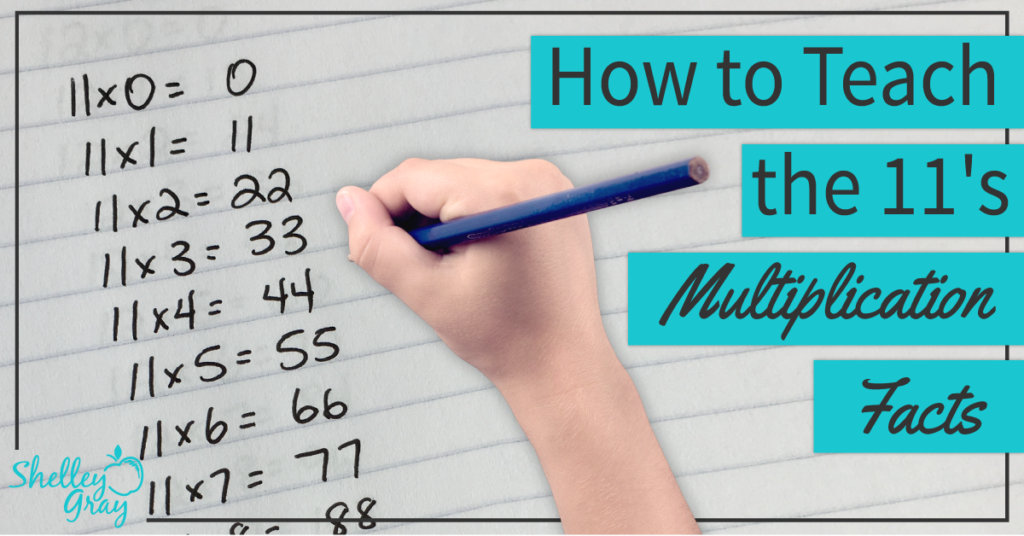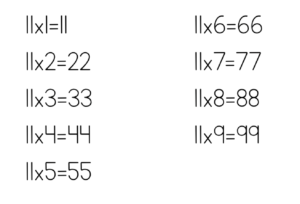# How to Teach the 11’s Multiplication FactsI like to teach the 11’s multiplication facts early on. This is because the 11’s facts from 0-9 are very simple.

For the 11’s facts, simply look at the other number (not the 11) and write that number twice. For example, 11×2 is 22, 11×5 is 55, 11×8 is 88.

When I first teach this trick, I like students to come up with the rule on their own. So I show them a list of all of the facts, and ask, “What do you notice?”Within a couple of minutes, most students will have realized the rule on their own.

This trick does not work for 11×10, 11×11, or 11×12, so you will need to teach these facts differently.

For 11×10, use the 10’s strategy of adding a 0. So you will add a 0 to the 11 to make 110.

For 11×11 and 11×12, you can use a known fact. For example, for 11×11, think, “10 groups of 11 is 110, and then one more group of 11 makes 121.” For 11×12, think, “10 groups of 11 makes 110, and two more groups of 11 makes 132.”

It’s also very important to reinforce the commutative property.

This means that the order of factors does not change the product. For example, if students are faced with an equation like 7×11, they should see the 11 as one of the factors, and know that they can use the 11’s strategy to solve this equation.

NEXT STEPS

Ready to tackle multiplication once and for all? Join me a free mini-course – “Teaching Basic Multiplication and Division for Mastery.” It’ll only take you about an hour to complete and you’ll leave with tons of great ideas, a game-plan, free resources, a PD certificate, and more! REGISTER HERE.Reinforce the 11’s multiplication facts with this set of task cards. Students will learn conceptually through problem-solving, using arrays, strategic thinking, finding missing numbers, skip-counting, picture representations, and more:OR FIND THE FULL BUNDLE OF MULTIPLICATION TASK CARDS HERE.